Most Affordable JEE | NEET | 8,9,10 Preparation by Kota's Top IITian Doctor Faculties

# p block Elements Class 11 Important Questions with Answers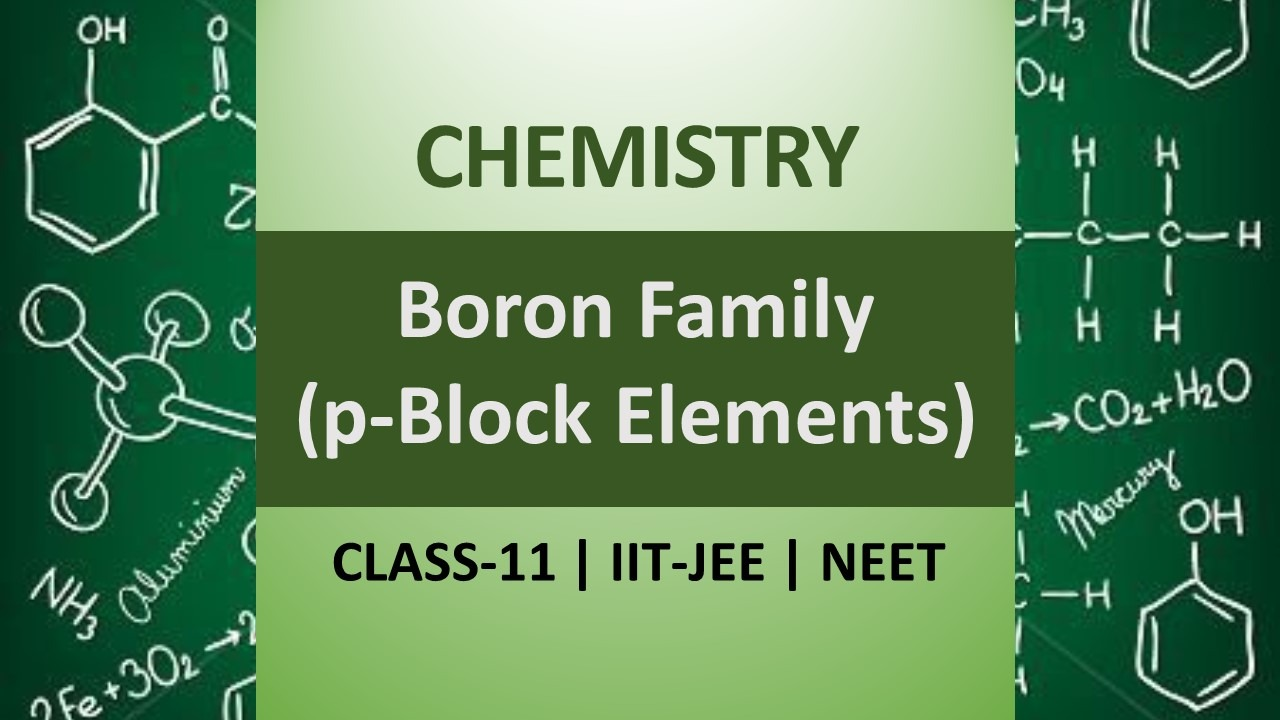Q. Write the electronic configuration of p-block elements.
Ans. Electronic configuration of $\mathrm{p}$ -block elements is $n s^{2} n p^{1-6}$
Q. Name the element having highest melting point.
Ans. The element having highest melting point is carbon (diamond).
Q. The element having highest melting point is carbon (diamond).
Ans. Amorphous and Crystalline.
Q. What is tincal ? Give its chemical formula.
Ans. Tincal is an ore of boron. Its formula is $\mathrm{Na}_{2} \mathrm{B}_{4} \mathrm{O}_{10} \cdot 10 \mathrm{H}_{2} \mathrm{O}$
Q. Why does boron not form $B^{3+}$ ions?
Ans. Boron has very small size and has very high sum of three ionisation enthalpies $\left(I E+I E_{2}+I E_{3}\right)$ therefore it cannot lose its three electrons to form $B^{3+}$ ions.
Q. Write the balanced equation for the preparation of elemental boron by reduction with dihydrogen.
Ans. $2 \mathrm{BCl}_{3}(\mathrm{g})+3 \mathrm{H}_{2}(\mathrm{g}) \frac{\mathrm{sun}}{_{1270 \mathrm{K}}} 2 \mathrm{B}(\mathrm{s})+6 \mathrm{HCl}(\mathrm{g})$
Q. Why is $B F_{3}$ weaker Lewis acid than $B C l_{3} ?$
Ans. $B F_{3}$ is weaker lewis acid than $B C l_{3}$ because of more effecttive back bonding in case of $F$ due to smaller size than $C l$
Q. Why does boric acid act as Lewis acid ?
Ans. It is because in boric acid, boron does not have its octet complete. It accepts $O H^{-}$ from water.
Q. Aluminium forms $\left[A I F_{6}\right]^{3-}$ but boron does not form $\left[B F_{6}\right]^{3-}$ Explain why?
Ans. Boron does not have vacant d-orbitals as second shell is the outer most shell.
Q. By means of a balanced chemical equation show how $B(O H)_{3}$ behaves as an acid in water. $\quad$ [NCERT]
Ans. $B(O H)_{3}$ is a weak monobasic acid. It acts as a lewis acid by accepting electrons from a hydroxy ion in water. $B(O H)_{3}+2 H O H \longrightarrow\left[B(O H)_{4}\right]^{-}+H_{3} O^{+}$
Q. How does sodium hydride react with diborane ?
Ans. Sodium borohydride is formed $2 \mathrm{NaH}+\mathrm{B}_{2} \mathrm{H}_{6} \stackrel{\text { heat }}{\longrightarrow} 2 \mathrm{NaBH}_{4}$
Q. What type of glass is obtained when borax is added ?
Ans. Pyrex glass is a glass which is heat resistant. It can with stand high temperature.
Q. Describe briefly how element boron can be prepared. [NCERT]
Ans. Boron can be obtained by reduction of Boron oxide with electropositive metal like Mg. $B_{2} O_{3}(s)+3 M g(g) \longrightarrow 2 B(s)+3 M g O(s)$
Q. What is the oxidation state of boron in boric acid ? What is the basicity of this acid ?
Ans. Boric acid is $H_{3} B O_{3}$ Oxidation state of boron $=+3$ Boric acid is tribasic.
Q. Boron is unable to form $B F_{6}^{3-}$ ion. Explain.
Ans. Boron does not have vacant $d$ -orbitals in its valence shell. Therefore, it cannot expand its octet. Therefore, maximum covalency of boron cannot exceed and it does not form $B F_{6}^{3-}$ ion.
Q. Why do boron halides form “addition compounds” with amines ?
Ans. Boron halides are electron deficient, therefore, they can form addition compounds with electron rich amines $e . g .$ $\left(\mathrm{CH}_{3}\right)_{3} \mathrm{N} \longrightarrow \mathrm{BF}_{3}$
Q. Describe what happens when boric acid is heated ?
Ans. When boric acid is heated at $370 K .$ metaboric acid $\left(H B O_{2}\right)$ is formed. On heating strongly $B_{2} O_{3}$ is formed. (i) $\mathrm{H}_{3} \mathrm{BO}_{3} \stackrel{370 \mathrm{K}}{\longrightarrow} \mathrm{HBO}_{2}+\mathrm{H}_{2} \mathrm{O}$ (ii) $2 H B O_{2} \stackrel{\text { heat }}{\longrightarrow} B_{2} O_{3}+H_{2} O$
Q. What happens when a borax solution is acidified ? Write a balanced equation of the reaction.
Ans. $\mathrm{Na}_{2} \mathrm{B}_{4} \mathrm{O}_{7} \cdot 10 \mathrm{H}_{2} \mathrm{O}+2 \mathrm{HCl}+5 \mathrm{H}_{2} \mathrm{O} \longrightarrow 2 \mathrm{NaCl}$ $+4 H_{2} B Q_{3}+12 H_{3} Q$
Q. Complete and balance the following chemical equation : (i) $B F_{3}+L i H \stackrel{450 K}{\longrightarrow}$ (ii) $\mathrm{B}_{2} \mathrm{H}_{6}+\mathrm{NaH} \longrightarrow$
Ans.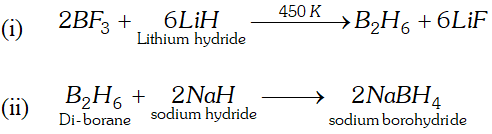Q. If $B-C l$ bond has a dipole moment, why does $B C l_{3}$ have zero dipole moment? [NCERT]
Ans. $=B-C l$ bond has dipole moment due to difference in electronegativity of $B$ and chlorine atom. $B C l_{3}$ on the contrary has a zero dipole moment because dipole moment is a vector quantity. The net resultant of the three bonds is zero due to its symmetrical planer triangular structure.
Q. Describe the shape of $B F_{3}$ and $B H_{4}^{-}$. Assign hybridisation of boron in these species. $\quad$ [NCERT]
Ans. - In $B F_{3}$ the hybridisation of boron is $s p^{2}$ and hence the molecule has a planar triangular shape. In $B H_{4}^{-}$ the hybridisation of boron is $s p^{3}$ and hence it has a tetrahedral shape.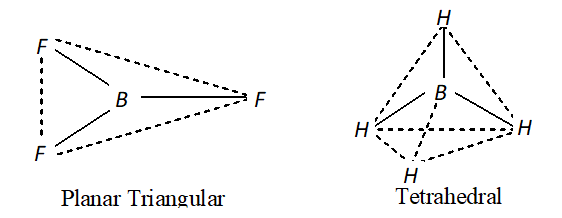Q. What are electron deficient compound? Are $B C l_{3}$ and $S i C l_{4}$ electron deficient species? Explain.
Ans. Electron deficient compounds are those which have incomplete octet. $B C l_{3}$ is an electron deficient compound as it has sextet of electrons. On the contrary $\mathrm{SiCl}_{4}$ is not an electron deficient compound. However, due to the presence of vacant $d$ -orbitals in its valence shell it can extend its co-ordination number and form species like $S i F_{3}^{-}, S i F_{6}^{2-},$ etc.
Q. Discuss the pattern of variation in oxidation state from $B$ to Tl in boron family. [NCERT]
Ans. - The boron family exhibits two oxidation states of $+3$ and $+1$ in their compounds on moving down the group from boron to thallium, the stability of lower oxidation state increases $i . e .,+1$ oxidation state becomes more prominent due to " inert pair effect?
Q. How can you explain higher stability of $B C l_{3}$ as compared to $T l C l_{3} ? \quad$ [NCERT]
Ans. $B C l_{3}$ has more stability than $T I C l_{3} .$ It is because boron due to its small size can exhibit only one oxidation state of $+3$ in its compounds. On the contrary. Thallium exhibits two oxidation states of $+3$ and $+1$ in its compounds. The lower oxidation state of $+1$ is more stable because of inert pair effect. To add further the increase in the magnitude of nuclear charge is not compensated by the increase in size as a result of which the $n s$ -electrons do not participate in bonding.
Q. How is lithium aluminium hydride (LiAlH_{4} ) ~ p r e p a r e d ? ~ W h a t ~ is its important use? [NCERT]
Ans. It is obtained by reacting $A I C I_{3}$ with $L i H$ in the presence of dry ether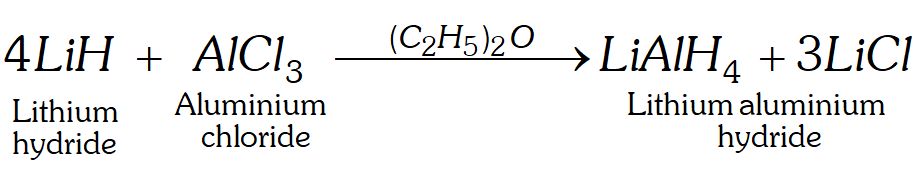Uses : It is a very good reducing agent used in many organic synthesis.
Q. Write balanced equation to show what happens when aq $\mathrm{NaOH}$ is added dropwise to aq $\mathrm{GaCl}_{3} .$ The precipitate formed initially dissolves on further addition of $N a O H$ solution. [NCERT]
Ans. $\mathrm{GaCl}_{3}+\mathrm{NaOH} \longrightarrow \mathrm{Ga}(\mathrm{OH})_{3}+3 \mathrm{NaCl}$ $\mathrm{Ga}(\mathrm{OH})_{3}+\mathrm{NaOH} \longrightarrow \underset{\text { Souble Complex }}{\mathrm{NaGaO}_{2}}+2 \mathrm{H}_{2} \mathrm{O}$
Q. Write the uses of boron compounds.
Ans. Uses of boron compounds : (i) The main use of borax and boric acid is in the preparation of heat resistant borosilicate glass such as pyrex glass. (ii) Borax is used as flux for soldering of metals and procelain enamels. (iii) Dilute aqueous solution of boric acid is used as mild antiseptic. (iv) Boron rods are used as control rods in nuclear reactors.
Q. How does boron react with (i) metals like $C r$ (ii) $N a O H$ (iii) acids like $H_{2} S O_{4}$ (conc.)?
Ans.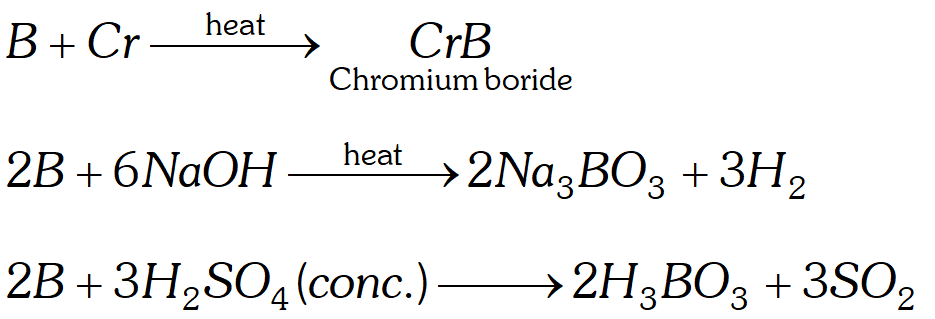Q. Write balanced equations for the reaction of elemental boron with elemental chlorine, oxygen and nitrogen at a high temperature. [NCERT]
Ans. (i) $\quad 2 B+3 C l_{2} \stackrel{\text { heat }}{\longrightarrow} 2 B C l_{3}$ (ii) $\quad 4 B+3 O_{2} \stackrel{\text { heat }}{\longrightarrow} 2 B_{2} O_{3}$ (iii) $\quad 2 B+N_{2} \stackrel{\text { heat }}{\longrightarrow} 2 B N$
Q. Suggest reason why $B-F$ bond length in $B F_{3}(130 p m)$ and $B F_{4}^{-}(143 p m)$ differ $?$ [NCERT]
Ans. $B-F$ bond length in $B F_{3}$ is 130 pm because boron in is hybridised and has a planar triangular structure. As a result of deficiency of electrons on the boron atom the excessive electron density on the fluorine atoms (due to its small size and strong interelectronic repulsions) is pushed on to the boron atom $i . e .$ back donation or back bonding occurs. This introduces a partially double bond character shortening the bond. However, in the boron atom is hybridised with bond length of $143 \mathrm{pm}$
Q. Why $B B r_{3}$ is a stronger Lewis acid as compared to $B F_{3}$ though fluorine is more electronegative than chlorine?
Ans. Both $B F_{3}$ and $B B r_{3}$ are electron deficient molecules due to presence of only six electrons around boron atom. Therefore, these molecules act as Lewis acids due to their tendency to accept a pair of electrons. In , the 2 p-orbital of adjoining $F$ atom containing lone pair of electrons form effective bonding with empty $2 \mathrm{p}$ -orbital of boron atom. As a result, the lone pair of $F$ is donated to $B$ atom and hence the electron-deficiency ofboron decreases. In contrast in, the size of $B r$ atom is much bigger than the $F$ atom, hence donation of lone pair of electrons of $B r$ to $B$ does not occur to any significant extent. As a result, the electron-deficiency of $B$ is much higher in $\mathrm{BBr}_{3}$ than that in $\mathrm{BF}_{3},$ and hence $\mathrm{BBr}_{3}$ is a stronger Lewis acid than $\mathrm{BF}_{3}$
Q. $A I F_{3}$ is insoluble in anhydrous $H F$ but dissolves on addition of $\mathrm{NaF.}$ Aluminium trifluoride precipitates when gaseous $B F_{3}$ is bubbled through it. Give reasons. $\quad$ [NCERT]
Ans.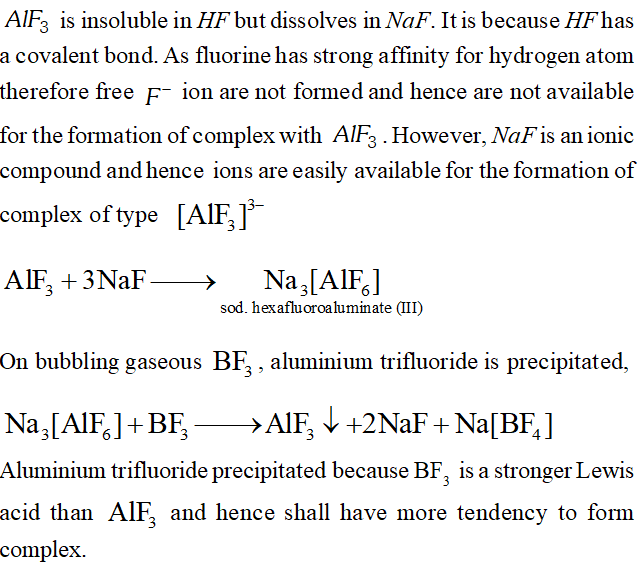Q. Give chemical equations to show the reaction between (i) Borax with ethanol in the presence of conc. $H_{2} \mathrm{SO}_{4}$ (ii) Diborane and chlorine (iii) Diborane and caustic potash (iv) Magnesium boride and phosphoric acid (v) Boron trifluoride and hydrofluoric acid.
Ans.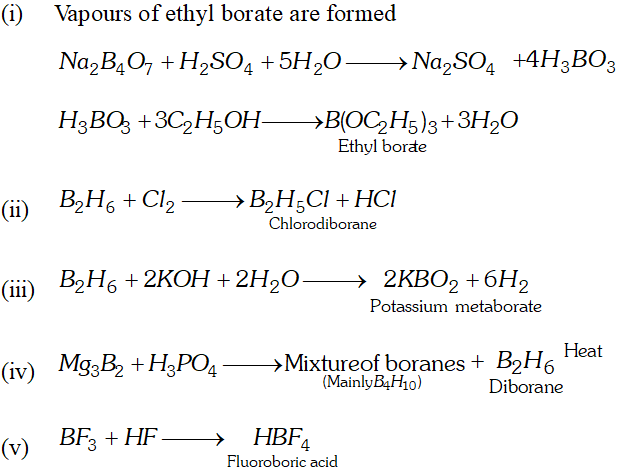Q. What is the structure of boric acid and what is its basicity? How can we get boric acid from, (i) colemanite (ii) borax? or What is the action of heat on boric acid ?
Ans. - Boric acid is $H_{3} B O_{3} .$ It can also be represented by $B(O H)_{3} .$ It has layer type structure in which $B O_{3}$ units are linked to one another through $H$ -bonds. The $H$ -atom constitutes covalent bond with one unit and $H$ -bond with other unit. Boric acid is a weak monobasic acid, it does not act as proton donor but as Lewis acid. $\mathrm{B}(\mathrm{OH})_{3}+\mathrm{H}_{2} \mathrm{O} \longrightarrow \mathrm{B}(\mathrm{OH})_{4}^{-}+\mathrm{H}^{+}$ Preparation (i) By the action of $\mathrm{SO}_{2}$ on boiling suspension of colemanite in water. $\mathrm{Ca}_{2} \mathrm{B}_{6} \mathrm{O}_{11}+2 \mathrm{SO}_{2}+9 \mathrm{H}_{2} \mathrm{O} \longrightarrow 2 \mathrm{CaSO}_{3}+6 \mathrm{H}_{3} \mathrm{BO}_{3}$ (ii) By acidifying aqueous solution of borax with mineral acids. $\mathrm{Na}_{2} \mathrm{B}_{4} \mathrm{O}_{7}+2 \mathrm{HCl}+5 \mathrm{H}_{2} \mathrm{O} \longrightarrow 2 \mathrm{NaCl}+4 \mathrm{H}_{3} \mathrm{BO}_{3}$ Effect of heat. Heating orthoboric acid above $370 K$ leads to partial removal of water to yield metaboric acid, $\left(\mathrm{HBO}_{2}\right) ;$ further heating yields.